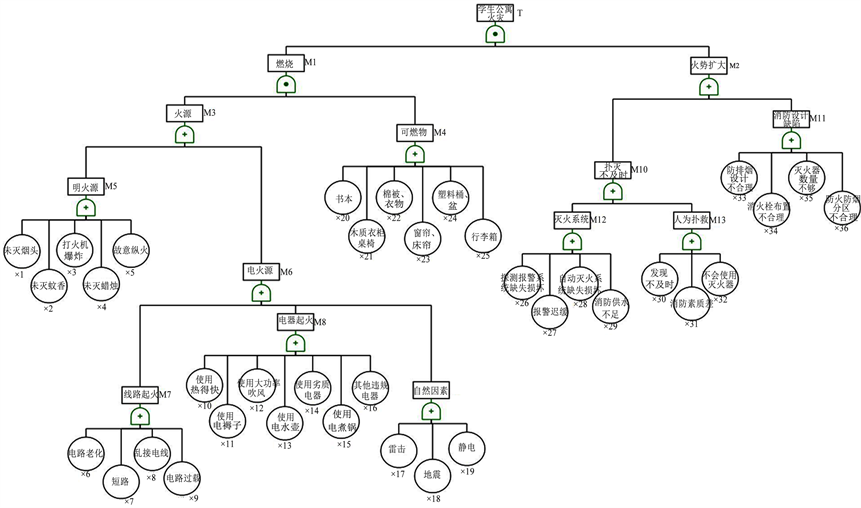# 基于事故树法的学生公寓火灾风险分析Fire Risk Analysis of Student Apartment Based on Accident Tree Method

DOI: 10.12677/JSST.2018.62005, PDF, HTML, XML, 下载: 688  浏览: 1,822  科研立项经费支持

Abstract: In view of the fire characteristic of fast fire spread and difficult evacuation in the student’s apartment, once the fire happens, it is prone to cause group death. The cause of the accident is summarized and analyzed by the investigation and analysis of the fire accident in the student’s apartment. The probability of the basic events is considered by the accident tree analysis (FTA) method, and qualitative and quantitative analysis was carried out. The minimum path set and the minimum cut set are calculated, and the risk is identified by the analysis of its structural importance, probability importance and critical importance. The ways to control the accident are found, and the prevention measures for such accidents are obtained to eliminate the fire hidden in the student’s apartment safety system. It has certain guiding significance for preventing similar fire accidents.

1. 引言

2. 事故树分析法基本理论

3. 学生公寓火灾事故致因因素的分析

3.1. 起火因素繁杂

3.2. 火势蔓延较快

4. 学生公寓火灾事故树分析

4.1. 学生公寓火灾伤亡事故树构建

4.2. 最小割集和最小径集的计算

$\begin{array}{c}\text{T}={\text{M}}_{\text{1}}\cdot {\text{M}}_{\text{2}}=\left({\text{M}}_{3}\cdot {\text{M}}_{4}\right)\left({\text{M}}_{10}+{\text{M}}_{11}\right)\\ =\left({\text{X}}_{1}+{\text{X}}_{2}+{\text{X}}_{3}+{\text{X}}_{4}+{\text{X}}_{5}+{\text{X}}_{6}+{\text{X}}_{7}+{\text{X}}_{8}+{\text{X}}_{9}+{\text{X}}_{10}+{\text{X}}_{11}+{\text{X}}_{12}+{\text{X}}_{13}\\ \text{\hspace{0.17em}}\text{\hspace{0.17em}}\text{ }+{\text{X}}_{14}+{\text{X}}_{15}+{\text{X}}_{16}+{\text{X}}_{17}+{\text{X}}_{18}+{\text{X}}_{19}\right)\cdot \left({\text{X}}_{20}+{\text{X}}_{21}+{\text{X}}_{2}{}_{2}+{\text{X}}_{23}+{\text{X}}_{24}+{\text{X}}_{25}\right)\\ \text{\hspace{0.17em}}\text{\hspace{0.17em}}\text{ }\cdot \left({\text{X}}_{26}+{\text{X}}_{27}+{\text{X}}_{28}+{\text{X}}_{29}+{\text{X}}_{30}+{\text{X}}_{31}+{\text{X}}_{32}+{\text{X}}_{33}+{\text{X}}_{34}+{\text{X}}_{35}+{\text{X}}_{36}\right)\end{array}$ (1)

{X1, X2, X3, X4, X5, X6, X7, X8, X9, X10, X11, X12, X13, X14, X15, X16, X17, X18, X19}, {X20, X21, X22, X23, X24, X25}, {X26, X27, X28, X29, X30, X31, X32, X33, X34, X35, X36}。

4.3. 结构重要度分析

I (X25) = I (X24) = I (X23) = I (X22) = I (X21) = I (X20) > I (X36) = I (X35) = I (X34) = I (X33) = I (X32) = I (X31) = I (X30) = I (X29) = I (X28) = I (X27) = I (X26) > I (X19) = I (X18) = I (X17) = I (X16) = I (X15) = I (X14) = I (X13) = I (X12) = I (X10) = I (X9) = I (X8) = I (X7) = I (X6) = I (X5) = I (X4) = I (X3) = I (X2) = I (X1)。Figure 1. Student apartment fire accident treeTable 1. Student apartment fire accident tree basic events

4.4. 概率重要度分析

$\text{Iq}=\frac{\partial \text{Q}}{\partial \text{qi}}$ (2)

Iq (X31) = 0.0899, Iq (X32) = Iq (X27) = 0.0889, Iq (X36) = Iq (X33) = Iq (X30) = 0.0881, Iq (X34) = Iq (X35) = Iq (X26) = Iq (X28) = Iq (X29) = 0.0872, Iq (X20) = Iq (X23) = 0.0855, Iq (X21) = 0.0846, Iq (X22) = Iq (X24) = 0.0837, Iq (X25) = 0.0829, Iq (X15) = 0.194, Iq (X12) = 0.192, Iq (X5) = Iq (X8) = Iq (X9) = Iq (X13) = Iq (X14) = Iq (X16) = 0.0190, Iq (X17) = I (X19) = 0.0188, Iq (X3) = Iq (X6) = Iq (X10) = Iq (X11) = Iq (X18) = 0.0186, Iq (X1) = Iq (X2) = Iq (X4) = Iq (X7) = 0.0184。

4.5. 临界重要度分析

$\text{Ic}=\frac{\partial \text{Q}/\partial \text{qi}}{\text{Q}/\text{qi}}$ (3)

Ic (X20) = Ic (X23) = 0.2091, Ic (X31) = 0.1759, Ic (X21) = 0.1655, Ic (X32) = Ic (X27) = 0.1306, Ic (X22) = Ic (X24) = 0.1286, Ic (X33) = Ic (X36) = Ic (X30) =0.0862, Ic (X25) =0.0810, Ic (X15) =0.0665, Ic (X12) = 0.0564, Ic (X5) = Ic (X8) = Ic (X9) = Ic (X13) = Ic (X14) = Ic (X16) = 0.0465, Ic (X34) = Ic (X35) = Ic (X28) = Ic (X29) = Ic (X26) = 0.0426, Ic (X17) = Ic (X19) = 0.0368, Ic (X3) = Ic (X6) = Ic (X10) = Ic (X11) =Ic (X18) = 0.0273, Ic (X1) = Ic (X2) = Ic (X4) = Ic (X7) = 0.0180。

5. 结论

1) 根据最小径集和结构重要度可知，降低学生公寓火灾事故发生的概率，应从消除火源、减少可燃物、控制火灾的蔓延三个方面入手。如减少可燃物可将木质的桌椅、衣柜用其他的材料代替，或在其表面涂上阻燃剂。Table 2. Student apartment fire accident tree basic event probability value

2) 概率重要度结果显示：建筑物的消防设计不合理、消防系统发生故障是导致学生公寓火灾事故的重要原因。因此在学生公寓建设初期应重视其消防设计，在建筑物投入使用期间定期对消防设施进行检修，可有效降低火灾发生的概率。

3) 根据临界重要度结果可知：学生在火灾事故中起着至关重要的作用。因此，学校应通过加强消防教育、定期安排消防演练等手段提高学生消防素质，使人人都掌握相应的灭火技能，学会使用灭火设备。

  贾水库, 田斌. 层次分析法在高层学生公寓火灾危险性评价中的应用[J]. 中国安全学报, 2009, 19(5): 114-118.  赵江平, 等. 高层学生公寓防火体系的构建[J]. 中国安全生产科学技术, 2012, 8(1): 97-102.  郑乐. 高层建筑火灾风险分析及对策研究[J]. 中国安全科学学报, 2009, 19(10): 72-76.  张光剑, 戴晓江, 李伟. 大型宾馆火灾事故树分析[J]. 安全工程与环境, 2007, 14(4): 83-85.  张村峰, 等.基于“事故树–层次分析法”的高校学生宿舍火灾风险分析[J]. 中国安全生产科学技术, 2011, 10(7): 100-105.  高云, 张浩. 高层建筑火灾致因因素分析与消防安全对策[J]. 中国安全科学学报, 2009, 19(6): 149-153.  刘辉, 等. 基于事故树法的高校学生宿舍火灾分析[J]. 吉林化工学院学报, 2014, 31(5): 76-79.  王泰升, 等. 用事故树分析(FTA)预防事故、分析事故[J]. 河北化工, 1989(3): 56-59.  尤立明, 等. 事故树分析在高校实验室消防安全管理中的应用[J]. 试验科学与技术, 2008, 25(6): 168-170.  王振, 刘茂. 应用区间层次分析法(IAHP)研究高层建筑火灾安全因素[J]. 安全与环境学报, 2006, 6(1): 12-15.  肖旻, 史毅, 等. 高校学生宿舍火灾荷载调查研究[J]. 北京建筑工程学院学报, 2009, 25(3): 27-31.  霍然, 胡源, 李元洲. 建筑火灾安全工程导论[M]. 合肥: 科技大学出版社, 2009.